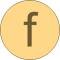# expression

Expression interpreter that returns with the position after the expression (expression may consist of +, -, *, /, (), sin(), cos(), tan(), sqrt(), asin(), acos(), atan(), exp(), log(), pi)# Information

This information is part of the Modelica Standard Library maintained by the Modelica Association.

#### Syntax

```             result = expression(string);
(result, nextIndex) = expression(string, startIndex=1, message="");
```

#### Description

This function is nearly the same as Examples.calculator. The essential difference is that function "expression" might be used in other parsing operations: After the expression is parsed and evaluated, the function returns with the value of the expression as well as the position of the character directly after the expression.

This function demonstrates how a simple expression calculator can be implemented in form of a recursive decent parser using basically the Strings.scanToken(..) and scanDelimiters(..) function. There are 2 local functions (term, primary) that implement the corresponding part of the grammar.

The following operations are supported (pi=3.14.. is a predefined constant):

```   +, -
*, /
(expression)
sin(expression)
cos(expression)
tan(expression)
sqrt(expression)
asin(expression)
acos(expression)
atan(expression)
exp(expression)
log(expression)
pi
```

The optional argument "startIndex" defines at which position scanning of the expression starts.

In case of error, the optional argument "message" is appended to the error message, in order to give additional information where the error occurred.

This function parses the following grammar

```  expression: [ add_op ] term { add_op term }
term      : primary { mul_op primary }
mul_op    : "*" | "/"
primary   : UNSIGNED_NUMBER
| pi
| ( expression )
| functionName( expression )
function  :   sin
| cos
| tan
| sqrt
| asin
| acos
| atan
| exp
| log
```

Note, in Examples.readRealParameter it is shown, how the expression function can be used as part of another scan operation.

#### Example

```  expression("2+3*(4-1)");  // returns 11
expression("sin(pi/6)");  // returns 0.5
```

# Syntax

(result, nextIndex) = expression(string, startIndex, message)

# Inputs (3)

string Type: String Description: Expression that is evaluated Default Value: 1 Type: Integer Description: Start scanning of expression at character startIndex Default Value: "" Type: String Description: Message used in error message if scan is not successful

# Outputs (2)

result Type: Real Description: Value of expression Type: Integer Description: Index after the scanned expression